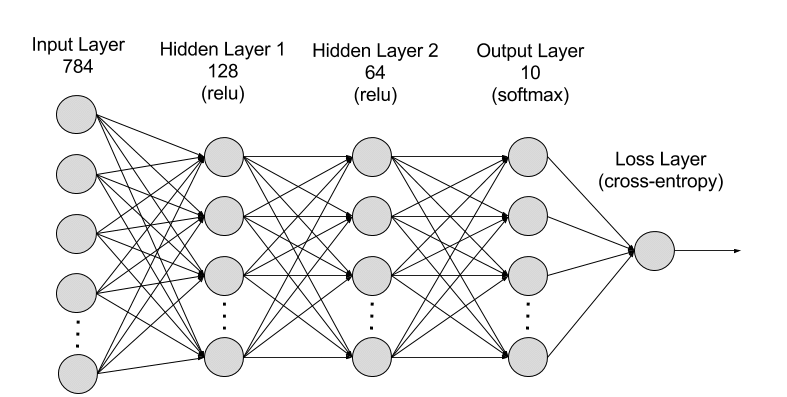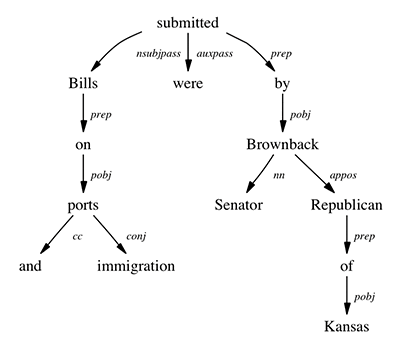# Introducing Gluon — An Easy-to-Use Programming Interface for Flexible Deep Learning

Today, AWS and Microsoft announced a new specification that focuses on improving the speed, flexibility, and accessibility of machine learning technology for all developers, regardless of their deep learning framework of choice. The first result of this collaboration is the new Gluon interface, an open source library in Apache MXNet that allows developers of all skill levels to prototype, build, and train deep learning models. This interface greatly simplifies the process of creating deep learning models without sacrificing training speed.

Here are Gluon’s four major advantages and code samples that demonstrate them:

## (1) Simple, easy-to-understand code

In Gluon, you can define neural networks using simple, clear, and concise code. You get a full set of plug-and-play neural network building blocks, including predefined layers, optimizers, and initializers. These abstract away many of the complicated underlying implementation details. The following example shows how you can define a simple neural network with just a few lines of code:

``````# First step is to initialize your model
net = gluon.nn.Sequential()
# Then, define your model architecture
with net.name_scope():
net.add(gluon.nn.Dense(128, activation="relu")) # 1st layer - 128 nodes
net.add(gluon.nn.Dense(64, activation="relu")) # 2nd layer – 64 nodes

The following diagram shows you the structure of the neural network:For more information, go to this tutorial to learn how to build a simple neural network called a multilayer perceptron (MLP) with the Gluon neural network building blocks. It’s also easy to write parts of the neural network from scratch for more advanced use cases. Gluon allows you to mix and match predefined and custom components in your neural network.

## (2) Flexible structure

Training neural network models is computationally intensive and, in some cases, can take days or even weeks. Many deep learning frameworks reduce this time by rigidly defining the model and separating it from the training algorithm. This rigid approach adds a lot of complexity and also makes debugging difficult.

The Gluon approach is different. It brings together the training algorithm and neural network model, thus providing flexibility in the development process without sacrificing performance. Central to this approach is the Gluon trainer method, which is used to train the model. The trainer method is dependent on the MXNet autograd library, which is used to automatically calculate derivatives (i.e., gradients). A derivate is a mathematical calculation measuring the rate of change for a variable. It is a necessary input for the training algorithm. The autograd library can efficiently implement these mathematical calculations and is essential for enabling the flexibility that Gluon offers. Now you can define a training algorithm that consists of a simple nested for loop by incorporating autograd and trainer.

``````epochs = 10

for e in range(epochs):
for i, batch in enumerate(train_data):
data = batch.data
label = batch.label
with autograd.record(): # Start recording the derivatives
output = net(data) # the forward iteration
loss = softmax_cross_entropy(output, label)
loss.backward()
trainer.step(data.shape)``````

This flexible structure makes your code intuitive and easy-to-debug, and opens the door for more advanced models. You can use familiar, native Python language constructs like a for loop or an if statement within your neural network or as part of your algorithm. By bringing the model and algorithm together every line of code within the model is executed, making it easier to identify the specific line of code causing a bug.

## (3) Dynamic graphs

In certain scenarios, the neural network model might need to change in shape and size during the training process. This is necessary in particular when the data inputs that are fed into the neural network are variable, which is common in Natural Language Processing (NLP) where each sentence inputted can be a different length. With Gluon, the neural network definition can be dynamic, meaning you can build it on the fly, with any structure you want, and using any of Python’s native control flow.

For example, these dynamic neural network structures make it easier to build a tree-structured Long Short-Term Memory (LSTM) model, which is a major development in NLP introduced by Kai Sheng Tai, Richard Socher, and Chris Manning in 2015. Tree LSTMs are powerful models that can, for example, identify whether a pair of sentences has the same meaning. Take the following example where both sentences essentially have the same meaning:

• “Michael threw the football in front of the player.”
• “The ball was thrown short of the target by Michael.”

It’s possible to just feed the sentences through a recurrent neural network (one popular sequence learning model) and make a classification. However, the main insight of tree LSTMs is that we often come at problems in language with prior knowledge. For example, sentences exhibit grammatical structure, and we have powerful tools for extracting this structure out of sentences. We can compose the words together with a tree-structured neural network whose structure mimics the known grammatical tree structure of the sentence, as the following diagram illustrates.(The Stanford Natural Language Processing Group)

This requires building a different neural network structure on the fly for each example. It is difficult to do with traditional frameworks, but Gluon can handle it without a problem. In the following code snippet, you can see how to incorporate a loop in each forward iteration of model training, and still benefit from the autograd and trainer simplifications. This enables the model to walk through the tree structure of a sentence and thus learn based on that structure.

``````def forward(self, F, inputs, tree):
children_outputs = [self.forward(F, inputs, child)
for child in tree.children]
#Builds the neural network based on each input sentence’s syntactic
#structure during the model definition and training process
…
``````

## (4) High performance

With the flexibility that Gluon provides, you can easily prototype and experiment with neural network models. Then, when speed becomes more important than flexibility (e.g., when you’re ready to feed in all of your training data), the Gluon interface enables you to easily cache the neural network model to achieve high performance and a reduced memory footprint. This only requires a small tweak when you set up your neural network after you are done with your prototype and ready to test it on a larger dataset. Instead of using Sequential (as shown earlier) to stack the neural network layers, you must use HybridSequential. Its functionality is the same as Sequential, but it lets you call down to the underlying optimized engine to express some or all of your model’s architecture.

``````net = nn.HybridSequential()
with net.name_scope():
net.add(nn.Dense(128, activation="relu")) # 1st layer - 128 nodes
net.add(nn.Dense(64, activation="relu")) # 2nd layer – 64 nodes
``````

Next, to compile and optimize HybridSequential, we can call its hybridize method:

``net.hybridize()``

Now, when you train your model, you will be able to get nearly the same high performance and reduced memory usage you get with the native MXNet interface.

## Getting started with Gluon

To start using Gluon, you can follow these easy steps for installing the latest version of MXNet, or you can launch the Deep Learning Amazon Machine Image (AMI) on the cloud. Next, we’ll walk through how to use the different components that we have discussed previously to build and train a simple two-layer neural network, called a multilayer perceptron. We recommend using Python version 3.3 or greater and implementing this example using a Jupyter notebook.

First, import MXNet and grab the gluon library in addition to the other required libraries, autograd and ndarray.

``````import mxnet as mx
from mxnet import gluon, autograd, ndarray``````

Then get the data and perform some preprocessing on it. We will import the commonly used MNIST dataset, which includes a large collection of images of handwritten digits and the correct labels for the images. We also reshape the pictures to an array to enable easy processing and convert the arrays to the MXNet native NDArray object class.

``````# Import the MNIST using a native MXNet utils function
data = mx.test_utils.get_mnist()

# Set up the training data and reshape the pictures
train_data = data['train_data'].reshape((-1, 784))
train_label = data['train_label']

# Set up the test data and reshape the pictures
test_data = data['test_data'].reshape((-1, 784))
test_label = data['test_label']

# Convert the data to NDArrays
train_data_mx = mx.nd.array(train_data)
train_label_mx = mx.nd.array(train_label)
test_data_mx = mx.nd.array(test_data)
test_label_mx = mx.nd.array(test_label)``````

Next, we create an iterator to hold the training data. Iterators are a useful object class for traversing through large datasets. Before doing so, we must first set the batch size, which defines the amount of data the neural network will process during each iteration of the training algorithm – in this case, 32.

``````batch_size = 32
train_data = mx.io.NDArrayIter(train_data_mx, train_label_mx, batch_size,
shuffle=True)           ``````

Now, we are ready to define the actual neural network. We will create two layers: the first will have 128 nodes, and the second will have 64 nodes. They both incorporate an activation function called the rectified linear unit (ReLU). Activation functions are important because they enable the model to represent non-linear relationships between the inputs and outputs. We also need to set up the output layer with the number of nodes corresponding to the total number of possible outputs. In our case with MNIST, there are only 10 possible outputs because the pictures represent numerical digits of which there are only 10 (i.e., 0 to 9).

``````# First step is to initialize your model
net = gluon.nn.Sequential()
# Then, define your model architecture
with net.name_scope():
net.add(gluon.nn.Dense(128, activation="relu")) # 1st layer - 128 nodes
net.add(gluon.nn.Dense(64, activation="relu")) # 2nd layer – 64 nodes

Prior to kicking off the model training process, we need to initialize the model’s parameters and set up the loss and model optimizer functions.

``````# We start with random values for all of the model’s parameters from a
# normal distribution with a standard deviation of 0.05
net.collect_params().initialize(mx.init.Normal(sigma=0.05))

# We opt to use softmax cross entropy loss function to measure how well the # model is able to predict the correct answer
softmax_cross_entropy = gluon.loss.SoftmaxCrossEntropyLoss()

# We opt to use the stochastic gradient descent (sgd) training algothrim
# and set the learning rate hyperparameter to .1
trainer = gluon.Trainer(net.collect_params(), 'sgd', {'learning_rate': .1})``````

Now it is time to define the model training algorithm. For each iteration, there are four steps: (1) pass in a batch of data; (2) calculate the difference between the output generated by the neural network model and the actual truth (i.e., the loss); (3) use autograd to calculate the derivatives of the model’s parameters with respect to their impact on the loss; and (4) use the trainer method to optimize the parameters in a way that will decrease the loss. We set the number of epochs at 10, meaning that we will cycle through the entire training dataset 10 times.

``````epochs = 10

for e in range(epochs):
for i, batch in enumerate(train_data):
data = batch.data
label = batch.label
with autograd.record(): # Start recording the derivatives
output = net(data) # the forward iteration
loss = softmax_cross_entropy(output, label)
loss.backward()
trainer.step(data.shape)``````

We now have a trained neural network model, so let’s see how accurate it is by using the test data that we set aside. We will compute the accuracy by comparing the predicted values with actual values.

``````acc = mx.metric.Accuracy()# Initialize accuracy metric
output = net(test_data_mx) # Run the test data through the neural network
predictions = ndarray.argmax(output, axis=1) # Predictions for test data
acc.update(preds=predictions, labels=test_label_mx) # Calculate accuracy
print(acc)
``````

To learn more about the Gluon interface and deep learning, you can reference this comprehensive set of tutorials, which covers everything from an introduction to deep learning to how to implement cutting-edge neural network models.Vikram Madan is a Senior Product Manager for AWS Deep Learning. He works on products that make deep learning engines easier to use with a specific focus on the open source Apache MXNet engine. In his spare time, he enjoys running long distances and watching documentaries.